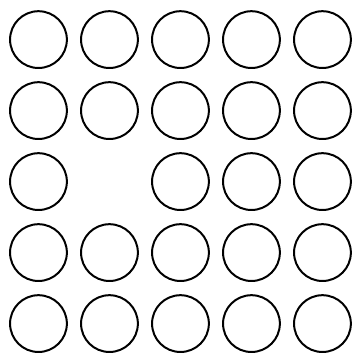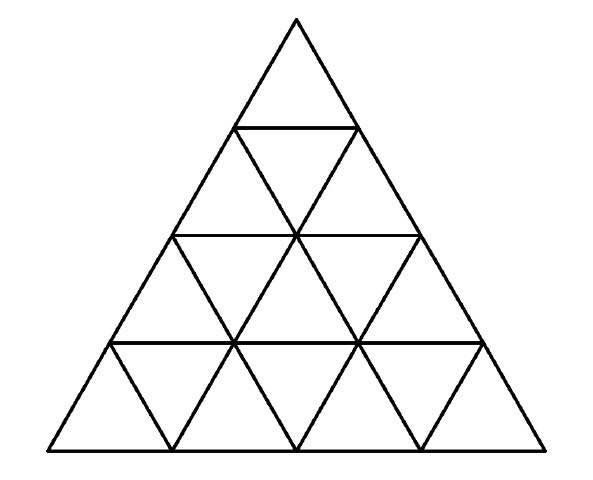###### back to index | new

There are $n$ points, $A_1$, $A_2$, $\cdots$, $A_n$ on a line segment, $\overline{A_0A_{n+1}}$. The point $A_0$ is black, $A_{n+1}$ is white, and the rest points are colored randomly either black or white. Prove: among these $n+1$ line segments $A_kA_{k+1}$, where $k=0, 1, \cdots, n$, the number of those with different colored ending points is odd.

$\textbf{Lily Pads}$

There are $24$ lily pads shown below. A toad can jump from one pad to an adjacent one either horizontally or vertically, but not diagonally. Can this toad visit all the pads without stopping at a pad for more than once? It can choose any pad to start its journey.As shown in the figure, in a regular triangular house, all the rooms are in the shape of regular triangles. There are doors between adjacent rooms. Starting from one of the rooms, go through the doors to visit other rooms, without repeating rooms or leaving the house. Including the starting room, how many rooms can be visited?Show that if an $m\times n$ grid can be completely covered by some L-shaped smaller grids consist of 4 unit grids without overlapping, then the value of $mn$ must be a multiple of 8.

In chess, a knight can move between the two opposite corner squares of a $2 \times 3$ block. The $2\times 3$ block can be either horizontal or vertical, and can be either direction of where the knight stands. In a $4\times 4$ grid, how many squares can a knight visit, including its starting square, in a series of moves without stopping at the same square twice?

Show that it is impossible to cover an $8\times 8$ square using fifteen $4\times 1$ rectangles and one $2\times 2$ square.

Is it possible to arrange these numbers, $1, 1, 2, 2, 3, 3, \cdots, 1986, 1986$ to form a sequence for such there is $1$ number between two $1$'s, $2$ numbers between two $2$'s, $\cdots$, $1986$ numbers between two 1986's?

It is possible to cover a $6\times 6$ grid using one L-shaped piece made of 3 grids and eleven $3\times 1$ smaller grid ?

$\textbf{Prisoners' Problem}$

One hundred prisoners will be lined up. Each one will be assigned either a red hat or a blue hat. No one can see the color of his or her own hat. However, each person is able to see the color of the hat worn by every person in front of him or her. That is, for example, the last person in line can see the colors of the hats on $99$ people in front of him or her; and the first person, who is at the front of the line, cannot see the color of any hat. Beginning with the last person in line, and then moving to the $99^{th}$ person, the $98^{th}$, etc., each will be asked to name the color of his or her own hat. He or she can only answer "red" or "blue". If the color is correctly named, the person lives; if not, the person is shot dead on the spot. Everyone in line is able to hear all the responses but not the gunshots. Before being lined up, the $100$ prisoners are allowed to discuss a strategy aiming to save as many of them as possible. How many people can be saved if they can agree on a good strategy?

As a more challenging question, what if the hats can have $100$ known different colors instead of $2$?

$\textbf{Coin Flipping}$

There are $9$ coins on the table, all heads up. In each operation, you can flip any two of them. Is it possible to make all of them heads down after a series of operations? If yes, please list a series of such operations. If no, please explain.